RY 48111 (099749002) - Ryobi Riding Mower, 48 Volt, Revision 04 (2017-11)

Riding Mower, 48 Volt, Revision 04

# RY 48111 (099749002) - Ryobi Riding Mower, 48 Volt, Revision 04 (2017-11) > Parts Diagrams (21) Hide 

•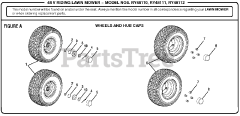Figure A

•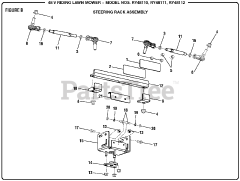Figure B

•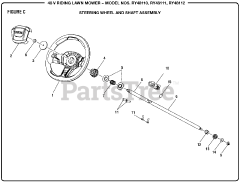Figure C

•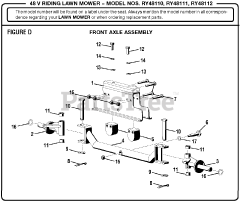Figure D

•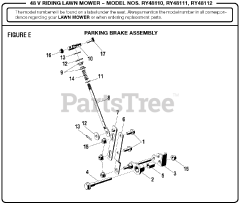Figure E

•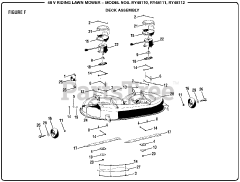Figure F

•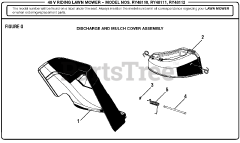Figure G

•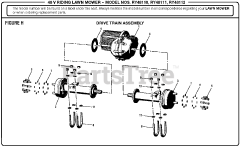Figure H

•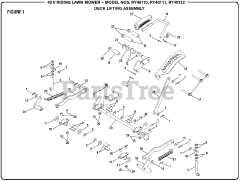Figure I

•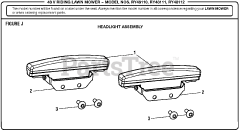Figure J

•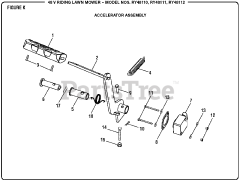Figure K

•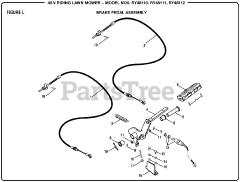Figure L

•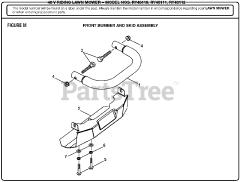Figure M

•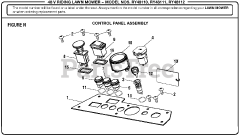Figure N

•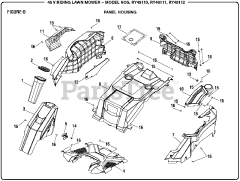Figure O

•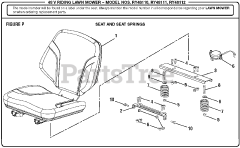Figure P

•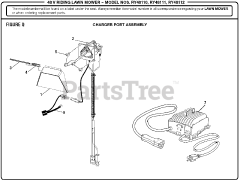Figure Q

•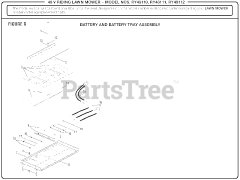Figure R

•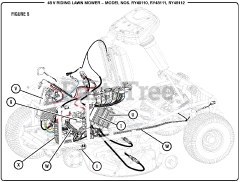Figure S

•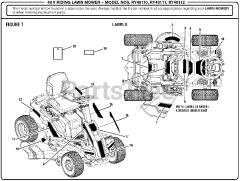Figure T

•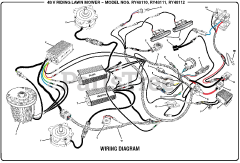Wiring Diagram